# [转载] $CF57D$ 题解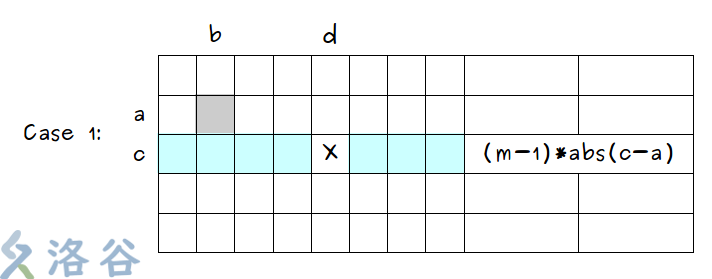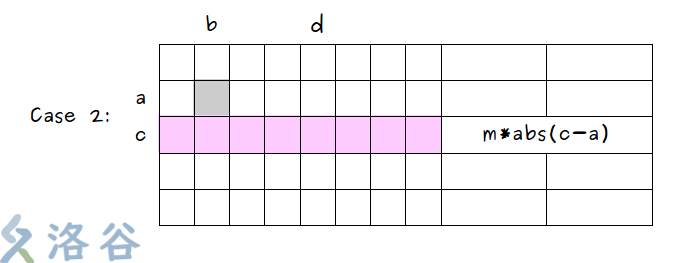#include<bits/stdc++.h> using namespace std; inline int read() { int ret=0,f=1; char ch=getchar(); while(ch>'9'||ch<'0') { if(ch=='-') f=-1; ch=getchar(); } while(ch>='0'&&ch<='9') { ret=(ret<<1)+(ret<<3)+ch-'0'; ch=getchar(); } return ret*f; } int n,m,totx,tot,hangx,liex; double ans; char s; inline long long finds(int n,int m,int a[]) { long long ret=0; for(register int i=1;i<=n;i++) { long long sum=0,cnt=m-a[i]; for(register int j=1;j<=n;j++) { if(a[j]) sum+=(m-1)*abs(i-j); else sum+=m*abs(i-j); } if(a[i]) ret+=(m-1)*sum; else ret+=m*sum; if(a[i]) { int l=i-1,r=i+1; while(a[l+1]<a[l]) { cnt+=m-a[l]; l--; } while(a[r-1]<a[r]) { cnt+=m-a[r]; r++; } ret+=4*cnt*(a[i]-1); } } return ret; } int main() { n=read(); m=read(); for(register int i=1;i<=n;i++) { scanf("%s",&s); for(register int j=1;j<=m;j++) { if(s[j-1]=='X') { hangx[i]=j; liex[j]=i; totx++; } } } tot=n*m-totx; ans=(finds(n,m,hangx)+finds(m,n,liex))*1.0/tot/tot; printf("%0.6lf\n",ans); return 0; }

##### 热门推荐
•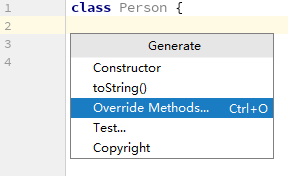浏览(613)
•浏览(399)
•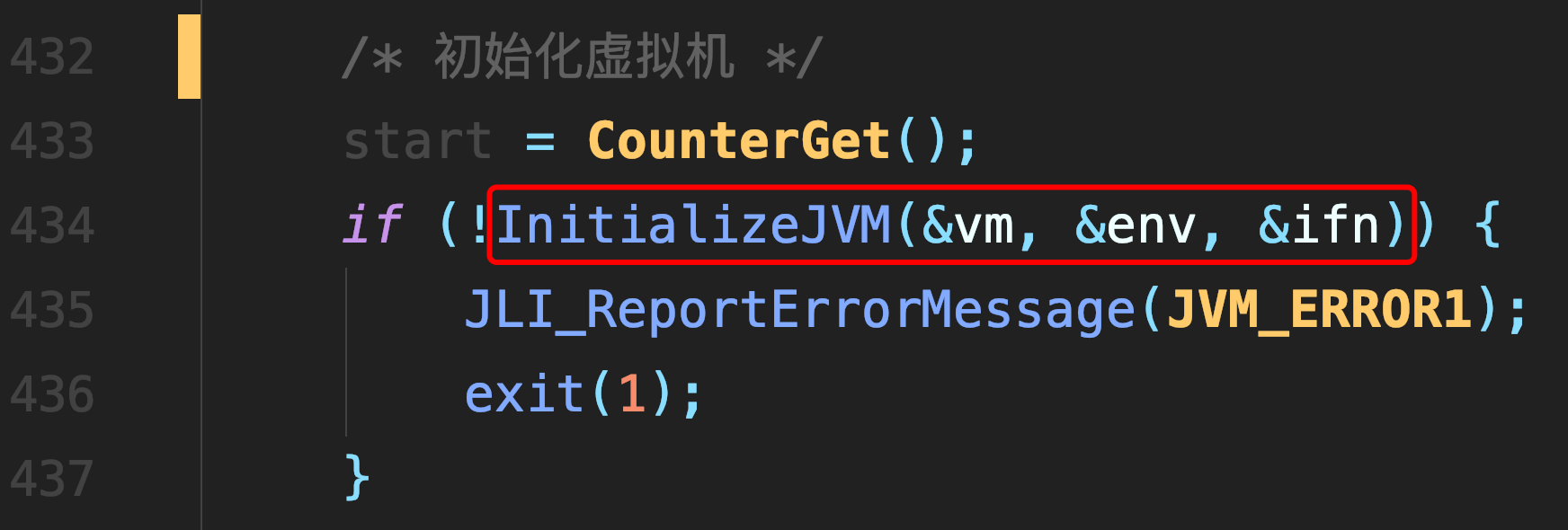浏览(365)
•浏览(359)
•浏览(347)
•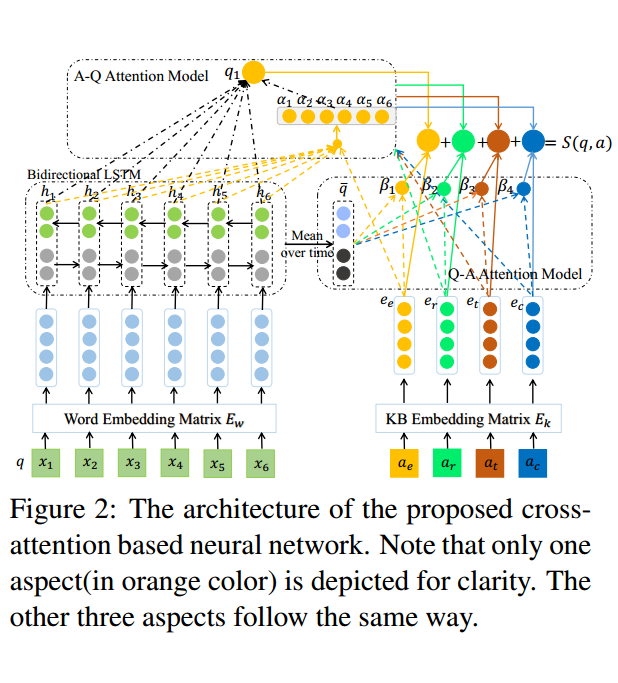浏览(318)
•浏览(295)
•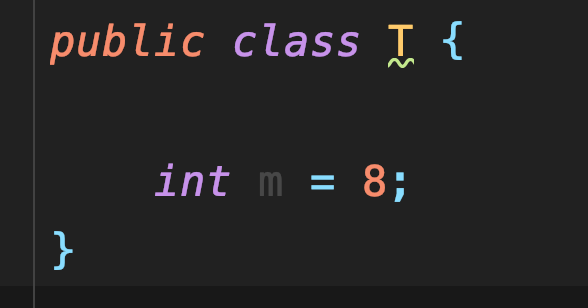浏览(281)
•浏览(275)
•浏览(251)